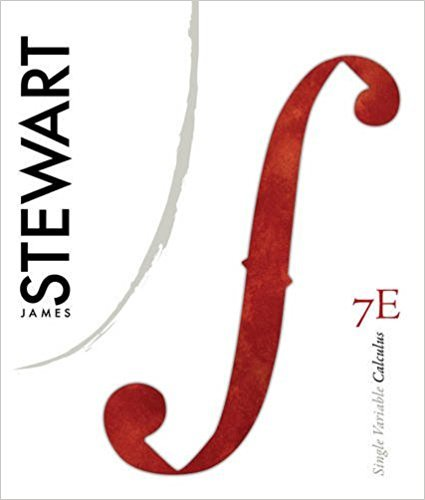×
Get Full Access to Single Variable Calculus - 7 Edition - Chapter 4 - Problem 14
Get Full Access to Single Variable Calculus - 7 Edition - Chapter 4 - Problem 14

×

# Evaluate the integral | Ch 4 - 14ISBN: 9780538497831 151

## Solution for problem 14 Chapter 4

Single Variable Calculus | 7th Edition

• Textbook Solutions
• 2901 Step-by-step solutions solved by professors and subject experts
• Get 24/7 help from StudySoup virtual teaching assistantsSingle Variable Calculus | 7th Edition

4 5 1 337 Reviews
11
1
Problem 14

Evaluate the integral

Step-by-Step Solution:
Step 1 of 3

Step 2 of 3

Step 3 of 3

##### ISBN: 9780538497831

The answer to “Evaluate the integral” is broken down into a number of easy to follow steps, and 3 words. Since the solution to 14 from 4 chapter was answered, more than 246 students have viewed the full step-by-step answer. The full step-by-step solution to problem: 14 from chapter: 4 was answered by , our top Calculus solution expert on 11/15/17, 04:21PM. Single Variable Calculus was written by and is associated to the ISBN: 9780538497831. This full solution covers the following key subjects: evaluate, integral. This expansive textbook survival guide covers 11 chapters, and 637 solutions. This textbook survival guide was created for the textbook: Single Variable Calculus, edition: 7.

Unlock Textbook Solution